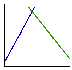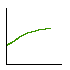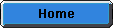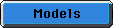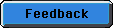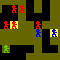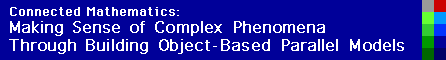Marketplace

This model illustrates the Law of Supply and Demand which is the fundamental tool of economic analysis. The law of supply and demand states that, in a free market, the price of a good will move to a point where the quantity supplied and the quantity demanded are equal. This point is known as 'equilibrium'.

This model demonstrates both the theory behind the law of supply and demand and how the law is realized in a theoretical market of buyers and sellers. The supply and demand functions can be computed and plotted ahead of time, thus yielding an equilibrium price as predicted by theory. The model can then be run, and from the interactions between the buyers and sellers we see that in trying to maximize profit the sellers eventually narrow in on the equilibrium price.

As the model is run it will iteratively cycle through two phases: an interaction phase (known as a 'round'), in which buyers visit sellers and purchase goods, and a adjustment phase, in which prices and inventories are redetermined and buyer and seller variables are reinitialized.

For detailed instructions on using the program, take a look at the info window from the Marketplace model.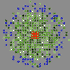The movie on the left shows buyers (in green) entering the marketplace (sellers are blue). 226K Sample plots of supply and demand curves, and average price over time are shown to the right.